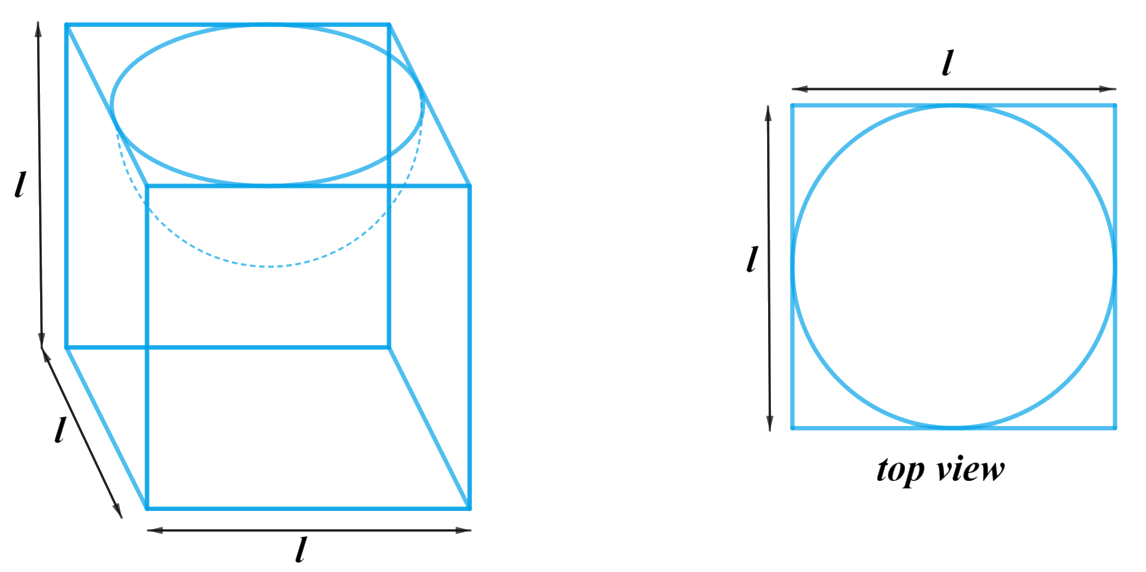# Ex.13.1 Q5 Surface Areas and Volumes Solution - NCERT Maths Class 10

Go back to  'Ex.13.1'

## Question

A hemispherical depression is cut out from one face of a cubical wooden block such that the diameter $$l$$ of the hemisphere is equal to the edge of the cube. Determine the surface area of the remaining solid.

Video Solution
Surface Areas And Volumes
Ex 13.1 | Question 5

## Text Solution

What is  known?

Diameter $$l$$ of the hemisphere is equal to the edge of the cube.

What is unknown?

The surface area of the remaining solid.

Reasoning:

We can create the figure of the solid as per given informationFrom the figure it’s clear that the surface area of the remaining solid includes TSA of the cube, CSA of the hemisphere and excludes base of the hemisphere.

Surface area of the remaining solid $$=$$ TSA of the cubical part$$+$$ CSA of the hemisphericalpart $$–$$ Area of the base of the hemispherical part

We will find the remaining area of the solid by using formulae;
TSA of the cube  $$= 6{l^2}$$
where $$l$$ is the length of the edge of the cube

CSA of the hemisphere  $$= 2\pi {r^2}$$
Area of the base of the hemisphere $$= \pi {r^2}$$
where $$r$$ is the radius of the hemisphere

Steps:

Diameter of the hemisphere $$=$$ Length of the edge of the cube  $$= l$$
Radius of the hemisphere,  $$r = \frac{l}{2}$$

Surface area of the remaining solid $$=$$ TSA of the cubical part $$+$$ CSA of the hemisphericalpart $$–$$ Area of the base of the hemispherical part

\begin{align}&= 6{l^2} + 2\pi {r^2} - \pi {r^2}\\&= 6{l^2} + \pi {r^2}\\&= 6{l^2} + \pi {\left( {\frac{l}{2}} \right)^2}\\&= 6{l^2} + \frac{{\pi {l^2}}}{4}\\&= \frac{1}{4}{l^2}\left( {\pi + 24} \right)\end{align}

Learn from the best math teachers and top your exams

• Live one on one classroom and doubt clearing
• Practice worksheets in and after class for conceptual clarity
• Personalized curriculum to keep up with school